Select Page

# Number concentration (num.c.)

Num.c.

### Definition in words

The number of component B divided by volume of system 1

### Definition by equation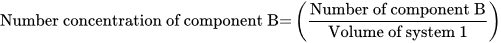### Systematic terms

Number concentration of component B in system 1

### Result type

Ratio scale
• Value sets are positive numerical values
• Algebraic operations are allowed (a=nb; a≠nb)
Numerical values from 0-∞

### Unit

1018/L, 1015/L, 1012/L, 109/L, etc.

### Reference

Ferard G, Dybkaer R, Fuentes-Arderiu X. Compendium of Terminology and Nomenclature of Properties in Clinical Laboratory Sciences : Recommendations 2016. 1 ed: Royal Society of Chemistry; 2016. 182 p.

### Clinical Chemistry

Example 1
Generic abbreviated
NPU01960 B—Erythrocytes; num.c. = ? × 1012/L
Full result NPU01960 Blood—Erythrocytes; number concentration = ? × 1012 per litre
Abbreviated result NPU01960 B—Erythrocytes; num.c. = ? × 1012/L
Written expression The number concentration of Erythrocytes in Mrs. Smith’s Blood is [4.8] × 1012/L.
Equation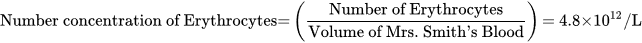### Clinical Immunology and Biobanking

Example 2
Generic abbreviated
NPU56976 BAL—T-lymphocytes(helper); num.c. = ? × 109/L
Full result NPU56976 Bronchoalveolar Lavage—T-lymphocytes(helper); number concentration = 7 × 109 per litre
Abbreviated result NPU56976 BAL—T-lymphocytes(helper); num.c. = 7 × 109/L
Written expression The number concentration of T-lymphocytes(helper) in Mrs. Smith’s Bronchoalveolar Lavage is  × 109/L.
Equation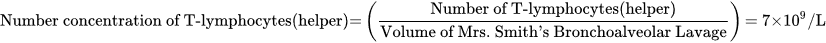### Clinical Microbiology

Example 3
Generic abbreviated
NPU17719 B—Loa loa; num.c. = ? × 103/L
Full result NPU17719 Blood—Loa loa; number concentration = 0 × 103 per litre
Abbreviated result NPU17719 B—Loa loa; num.c. = 0 × 103/L
Written expression The number concentration of Loa loa in Mr. Smith’s Blood is  × 103/L.
Equation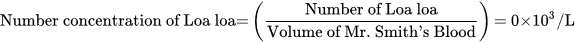### Clinical Toxicology

Example 4
Generic abbreviated
NPU16536 BAL—Asbestos fibres(length >5 µm; aspect ratio(L:D)>= 3:1); num.c.(proc.) = ? × 1/L
Full result NPU16536 Bronchoalveolar Lavage—Asbestos fibres(length >5 µm; aspect ratio(L:D)>= 3:1); number concentration(procedure) = 0 × 1 per litre
Abbreviated result NPU16536 BAL—Asbestos fibres(length >5 µm; aspect ratio(L:D)>= 3:1); num.c.(proc.) = 0 × 1/L
Written expression The number concentration of Asbestos fibres(length >5 µm; aspect ratio(L:D)>= 3:1) in Mr. Smith’s Bronchoalveolar Lavage is  × 1/L. The measurement is performed according to a procedure defined be the laboratory.
Equation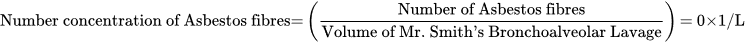Notes The Asbestos fibres are categorized according to the specified information: “length >5 µm; aspect ratio(L:D)>= 3:1.” (These information is not included in the equation due to technical reason)

### Molecular Biology and Genetics

Example 5
Generic abbreviated
NPU54794 B—ABL1/BCR Fusion Gene(RNA); num.c. = ? × 103/L
Full result NPU54794 Blood—ABL1/BCR Fusion Gene(RNA); number concentration = 25 × 103 per litre
Abbreviated result NPU54794 B—ABL1/BCR Fusion Gene(RNA); num.c. = 25 × 103/L
Written expression The number concentration of ABL1/BCR Fusion Gene(RNA) in Mrs. Smith’s Blood is  × 1/L. The measurement is performed according to a procedure defined be the laboratory.
Equation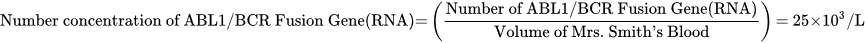### Reproduction and Fertility

Example 6
Generic abbreviated
NPU03455 Sem—Spermatozoa; num.c. = ? × 109/L
Full result NPU03455 Semen—Spermatozoa; number concentration = 30 × 109 per litre
Abbreviated result NPU03455 Sem—Spermatozoa; num.c. = 30 × 109/L
Written expression The number concentration of Spermatozoa in Mr. Smith’s Semen is  × 109/L.
Equation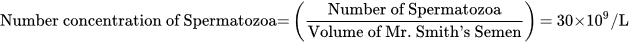### Reference

NPU database, https://www.ifcc.org/ifcc-scientific-division/sd-committees/c-npu/npusearch/ and http://www.labterm.dk/default.aspx
• # Kind-of-property

• ## Kind-of-quantity

### Reference

• Dybkaer R. Concept system on ‘quantity’: formation and terminology. Accredit Qual Assur. 2013;18(3):253-60.
• Dybkaer R. ISO terminological analysis of the VIM3 concepts ‘quantity’ and ‘kind-of-quantity’. Metrologia. 2010;47(3):127-34.
Date Term Note
1996-01-01 Number concentration Term established

QU50043